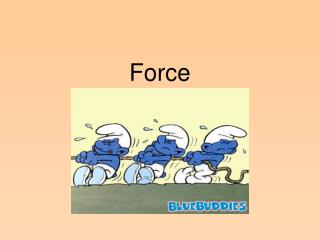DownloadDownload PresentationForce

# Force

Download Presentation## Force

- - - - - - - - - - - - - - - - - - - - - - - - - - - E N D - - - - - - - - - - - - - - - - - - - - - - - - - - -
##### Presentation Transcript

1. Force

2. Force Defined • A ________ or _______. (an action that has the ability to change motion) • Causes objects to: • Start moving • ______________ up/slow down • Change _____________ • Measured in Newtons (N) with a spring scale (1 lb. = 4.448 newtons)

3. Types of Force • Net Force-(FN) • Involves a ___________ of forces • It is the overall or __________ force • Effort / Applied Force (FEor FA )- the push or pull ________ to an object • Tension- ___________ force

4. Types of Force, cont. • Gravitational (gravity)- force of __________ between objects. • Weight (another force): the amount gravity pulling on a ___________. http://www.exploratorium.edu/ronh/weight - Increase mass = increase weight • Decrease distance = increase attraction (gravity) = increase in weight • Support - (Fs) • _________opposite to the force of gravity • Also called the normal force • Centripetal- (will talk about this later)

5. Types of Force, cont. • Electrical / “electromotive force (emf)” – • Emf: the force that ________ electrons to make electricity • Electrical- opposite charges attract and same charges repel • Magnetic- opposites poles of a magnet attract; same poles repel • Friction (FFr)- force that acts in a direction ______________ to applied force.

6. Friction(see handout) • For an object to move the applied force must be _________ than the force of friction • Types of friction: • Sliding • Rolling • Fluid • Static • The amount of friction depends on the contact between materials

7. Balanced and Unbalanced Forces(see handout) • Balanced – forces acting in OPPOSITE directions • Unbalanced- forces acting in the SAME direction

8. Centripetal Force “aiming toward the center” • For objects in circular motion • Force is pointed or directed _________ the __________

9. Projectile Motion • Projectile- any object ____________ or thrown into the air. • Projectile is affected by two things: • ______________ motion and velocity = object continues in a straight path due to its inertia • ______________ motion and velocity= object is pulled downward due to the force of gravity • Horizontal and vertical motion create a __________ path called a trajectory • (see hand out for picture)

10. Kepler’s Laws of Planetary Motion • Johannes Kepler developed 3 laws of planetary motion • 1st Law= planets ___________ around the sun in the shape of an ____________ with sun at one focus (or center).

11. Bernoulli’s Principle • As the speed in a fluid increases the pressure in that fluid decreases. • Creates “lift” for an airplane or your ping pong ball!

12. Archimedes Principle • Upward force acting on a fluid is called the buoyant force. • For liquids this force is made by the amount of liquid displaced by an object. • Buoyant force >equal to object’s weight the object floats. • Buoyant force < object’s weight the object sinks.